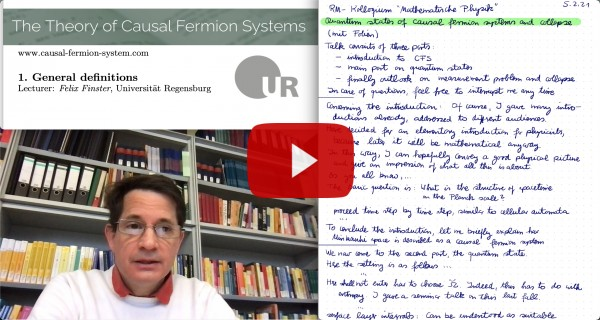# The Theory of Causal Fermion Systems

## Construction of Linear Fields and WavesThe existence theory for solutions of the linearized field equations and of the dynamical wave equation can be developed based on positivity properties of surface layer integrals. We outline the methods and results in different cases:

## The Linearized Field Equations in the Time-Dependent Setting

The existence theory for the linearized field equations is developed in [linhyp18]. The method is inspired by energy estimates for symmetric hyperbolic systems as used in the theory of hyperbolic partial differential equations. The methods are based on positivity properties of so-called softened surface layer integrals of the form

$\int_U d\rho(x)\: \eta_t(x) \int_U d\rho(y)\: \big(1-\eta_t(y)\big)\: \Big( \nabla_{1,\mathfrak{v}}^2 – \nabla_{2,\mathfrak{v}}^2 \Big) \L(x,y) \:,$

where $\eta_t$ is a smooth cutoff function in time, and $U \subset M$ is a so-called lens-shaped region. The time function and the lens-shaped region must satisfy hyperbolicity conditions. Under these assumptions, one obtains global existence of solutions of the Cauchy problem and finite propgation speed. The solutions are unique up to jets in the orthogonal complement of the test jets.

More recently, in [FK22] a method was developed for constructing global solutions by “glueing together” solutions obtained locally in lens-shaped regions.

## The Linearized Field Equations in the Static Setting

For static causal fermion systems, the existence theory as developed in [static21] is inspired by methods of elliptic partial differential equations. The starting point are the positive functionals obtained from second variations of the causal action. The linearized field equations can be formulated weakly with an integral operator which is bounded and symmetric on a Hilbert space endowed with a suitably adapted weighted $L^2$-scalar product. Using the spectral calculus of this operator, one can define Sobolev-type Hilbert spaces and invert the linearized field operator as an operator between such function spaces.

## The Dynamical Wave Equation

The existence theory for the dynamical wave equation is developed in [FKO21]. Similar as for the linearized field equations, the method is to use energy estimates as used for hyperbolic partial differential equations in the theory of symmetric hyperbolic systems. The methods are based on positivity properties of the softened surface layer integral

\begin{align*}
\la \psi | \phi \ra^t_\rho = -2i \int_M d\rho(x) \int_M d\rho(y) \:
\Big( \eta_t(x)\: \big( 1 &-\eta_t(y) \big) – \big(1-\eta_t(x) \big)\:\eta_t(y) \Big) \\
&\times \Sl \psi(x) \:|\: Q^\text{dyn}(x,y)\, \phi(y) \Sr_x \:,
\end{align*}

where $\eta_t$ is a smooth cutoff function in time. Assuming that such smooth cutoff functions exist with the property that suitable hyperbolicity conditions hold, one obtains global existence of solutions of the Cauchy problem and finite propgation speed. The solutions are again unique up to jets in the orthogonal complement of the test jets.

## Computational Approach in Minkowski Space

Based on the computations in [pfp06, cfs16], in [F23] the linearized field equations are analyzed systematically for causal fermion systems in Minkowski space. Using methods of functional analysis and Fourier analysis and making use of a direction-dependent local phase freedom, it is shown that the linearized field equations admit a multitude of homogeneous solutions. The time evolution of the inhomogeneous equations leads to the dynamical creation of retarded solutions as well as to the generation of non-propagating perturbations.

These results are the starting point for the detailed computational analysis of the causal action principle.